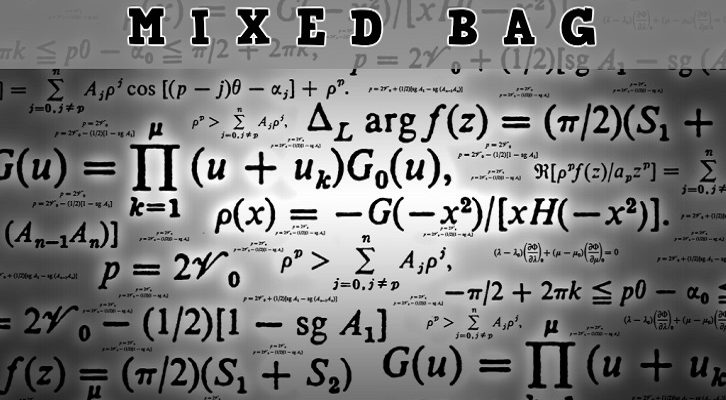# CAT Functions – Answers

Answers to questions on Functions posted more than a while ago here

1. Give the domain and range of the following functions

i. f(x) = x2 + 1
Domain = All real numbers (x can take any value)
Range  [1, infinity). Minimum value of x2 is 0.

ii. g(x) = log (x + 1)
Domain = Log of a negative number is not defined so (x + 1) > 0 or x > -1
Domain ( -1, infinity)
Range = (-infinity, +infinity)
Note: Log is one of those beautiful functions that is defined from a restricted domain to all real numbers. Log 0 is also not defined. Log is defined only for positive numbers
iii. h(x) = 2xDomain – All real numbers.
Range = (0, infinity)
The exponent function is the mirror image of the log function.
iv. f(x) = 1/ (x+1)
Domain = All real numbers except -1
Range = All real numbers except 0
v. p(x) = | x + 1|
Domain = All real numbers
Range = [0, infinity) Modulus cannot be negative
vi. q(x) = [2x], where [x] gives the greatest integer less than or equal to x
Domain = All real numbers
Range = All integers
The range is NOT the set of even numbers. [2x] can be odd. [2*0.6] = 1. It is very important to think fractions when you are substituting values.
Best wishes for CAT 2012.